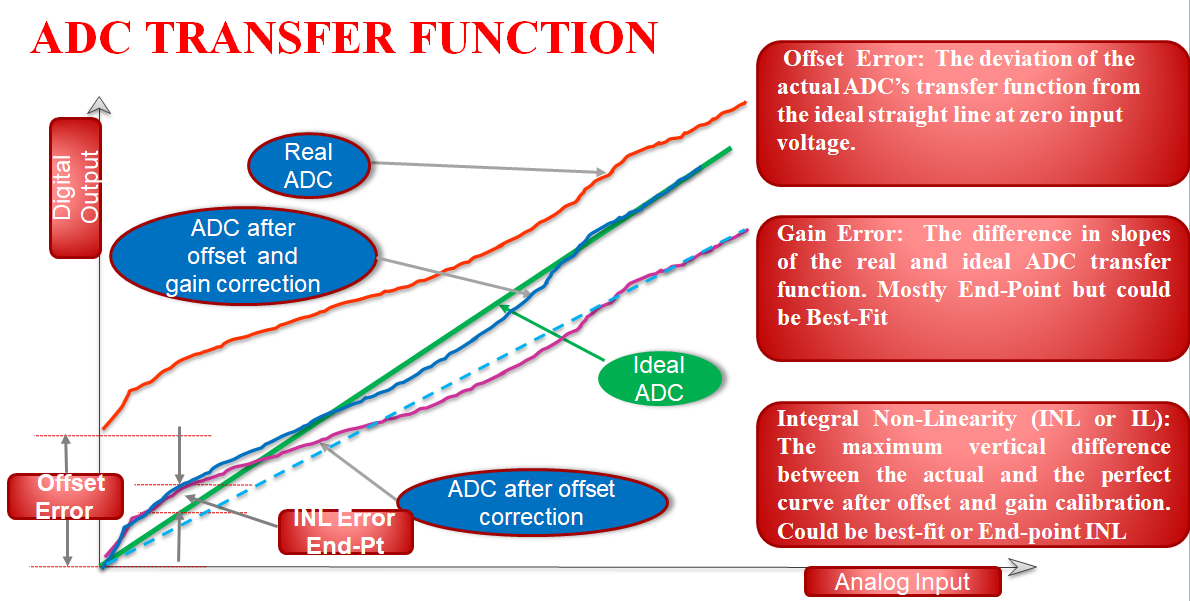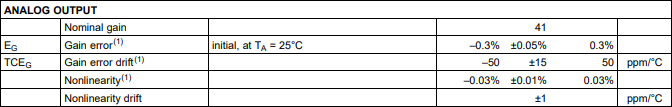If you have a related question, please click the "Ask a related question" button in the top right corner. The newly created question will be automatically linked to this question.

AMC1302-Q1: What do "TCEG Nonlinearity" and "TCEG Nonlinearity drift" mean?

Part Number: AMC1302-Q1

Hi team,

I found TCEG, TCEG Nonlinearity, TCEG Nonlinearity drift in the datasheet.

Could you show me the relationship among them? Could you show me an example characteristics between temperature vs gain error or TCEG?

Regards,
Ochi

• Hi Ochi-san,

The transfer function for an ADC can be shown in terms of y=mx+b, where the x axis is the input voltage and the y axis is the corresponding output voltage. There are three main terms that describe the accuracy of this transfer function. Offset, gain, and nonlinearity. Each of these terms also have a corresponding drift specification to define how these terms change in response to changes in temperature.

Offset is the "b" term from the y=mx+b equation.

Gain is the "m" term from the y=mx+b equation.

Nonlinearity accounts for the errors not represented by the y=mx+b equation. Often caused by the complex inner-workings of the device.

Below shows an example transfer function.

Red shows the real raw output transfer function without any calibration, while green shows the ideal transfer function, what the output would be if the ADC was perfect.

Purple shows the output transfer function after performing an offset calibration. This can be accomplished by shorting the inputs of the ADC together, recording the output result, then subtracting that result from the raw output.

Blue shows the output transfer function after performing a gain and offset calibration. Gain calibration can be performed by forming a line of best fit to the actual output (shown as the dotted light blue line) and comparing this line of best fit to the ideal output.

The variation between the green "ideal ADC" line and the blue "ADC after offset and gain correction" is the nonlinearity.From the AMC1302-Q1 datasheet:TCEG stands for "temperature coefficient Error Gain" Also known as gain error drift. TCEG does not apply to nonlinearity and nonlinearity drift.